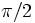# Linear representation theory of dihedral group:D8

View linear representation theory of particular groups | View other specific information about dihedral group:D8

The dihedral group of order eight is an example of a rational-representation group: all its faithful irreducible representations over the complex numbers can be realized over the rational numbers.

The character table of the dihedral group is the same as that of the quaternion group (get more information on the linear representation theory of quaternion group).

We shall use the dihedral group with the following presentation:$\langle a,x \mid a^4 = x^2 = e, xax^{-1} = a^{-1} \rangle$.

## Representations

### Trivial representation

The trivial representation is a one-dimensional representation that sends all elements of the dihedral group to$1$.

### Sign representations with kernels as the maximal normal subgroups

The dihedral group has three normal subgroups of index two: the subgroup$\langle a \rangle$, the subgroup$\langle a^2, x \rangle$, and the subgroup$\langle a^2, ax \rangle$. For each such subgroup, there is an irreducible one-dimensional representation sending elements in that subgroup to$1$ and elements outside that subgroup to$-1$.

The trivial representation and the three sign representations described above are the only faithful one-dimensional representations.

### Two-dimensional irreducible representation

The dihedral group of order eight has a two-dimensional irreducible representation, where the element$a$ acts as a rotation (by an angle of$\pi/2$, and the element$x$ acts as a reflection about the first axis. The matrices are:$a \mapsto \begin{pmatrix}0 & -1 \\ 1 & 0 \\\end{pmatrix}, \qquad x \mapsto \begin{pmatrix}1 & 0 \\ 0 & -1 \\\end{pmatrix}.$

There are many other choices of two-dimensional representation, but these are all equivalent as linear representations.

### Over other fields

These representations generalize to any field of characteristic not equal to$2$.

## Character table

This character table works over characteristic zero:

Rep/Conj class$e$$a^2$$\{ a, a^{-1} \}$$\{ x, a^2x \}$$\{ ax, a^3x \rangle$
Trivial representation 1 1 1 1 1$\langle a \rangle$-kernel 1 1 1 -1 -1$\langle a^2, x \rangle$-kernel 1 1 -1 1 -1$\langle a^2, ax\rangle$-kernel 1 1 -1 -1 1
2-dimensional 2 -2 0 0 0

## Realizability information

### Smallest ring of realization

Representation Smallest ring over which it is realized Smallest set of elements in matrix entries
trivial representation$\mathbb{Z}$ -- ring of integers$\{ 1 \}$$\langle a \rangle$-kernel$\mathbb{Z}$ -- ring of integers$\{ 1, -1 \}$$\langle a^2,x \rangle$-kernel$\mathbb{Z}$ -- ring of integers$\{ 1,0,-1 \}$

### Smallest ring of realization as orthogonal matrices

Representation Smallest ring over which it is realized Smallest set of elements in matrix entries
trivial representation$\mathbb{Z}$ -- ring of integers$\{ 1 \}$$\langle a \rangle$-kernel$\mathbb{Z}$ -- ring of integers$\{ 1, -1 \}$$\langle a^2,x \rangle$-kernel$\mathbb{Z}$ -- ring of integers$\{ 1,0,-1 \}$

## Orthogonality relations and numerical checks

• Any field of characteristic not equal to two is a splitting field for the dihedral group.
• The number of irreducible representations over such a field is$5$, which is equal to the number of conjugacy classes.
• The number of one-dimensional representations equals$4$, which is the order of the abelianization.
• The sum of squares of degrees is$1^2 + 1^2 + 1^2 + 1^2 + 2^2 = 8$, equal to the order of the group. This confirms the fact that sum of squares of degrees of irreducible representations equals order of group.
• The degrees of irreducible representations all divide$2$, which is the smallest possible index of an abelian normal subgroup. This confirms the fact that degree of irreducible representation divides index of abelian normal subgroup.
• The order of the inner automorphism group (which is$4$) bounds the square of the degree of every irreducible representation. This confirms the fact that order of inner automorphism group bounds square of degree of irreducible representation.
• The character table satisfies the orthogonality relations: in particular, the row orthogonality theorem and the column orthogonality theorem.

## Action of automorphism group

The automorphism group of the dihedral group preserves the trivial representation, the two-dimensional representation, and the sign representation whose kernel is the cyclic group$\langle a \rangle$. The two sign representations with kernels$\langle a^2, x \rangle$ and$\langle a^2,ax\rangle$ are exchanged by an outer automorphism.

## Relation with representations of subgroups

### Induced representations from subgroups

Since the dihedral group is a finite nilpotent group, it is in particular a finite supersolvable group, and hence, it is a monomial-representation group: every irreducible representation can be realized as a monomial representation, i.e., every irreducible representation is induced from a degree one representation of a subgroup. (Point (5) below explains how the two-dimensional irreducible representation is induced).

1. The trivial representation on the center induces a representation obtained as a sum of the four one-dimensional representations.
2. The sign representation on the center (which comprises$\pm 1$) induces the double of the two-dimensional irreducible representation of the dihedral group.
3. The trivial representation on the cyclic subgroup generated by$a$ induces a representation on the whole group that is the sum of a trivial representation and the representation with the$a$-kernel.
4. A representation on$\langle a \rangle$ that sends$a$ to$-1$ induces a representation of the whole group that is the sum of the sign representations for the other two kernels.
5. A representation on$\langle a \rangle$ that sends$a$ to$i$ (now viewed as a complex number) induces the two-dimensional irreducible representation.

### Verification of Artin's induction theorem

Artin's induction theorem states that the characters induced from characters on cyclic subgroups span the space of class functions. Points (2) and (5) cover the case of the two-dimensional irreducible representation. PLACEHOLDER FOR INFORMATION TO BE FILLED IN: [SHOW MORE]